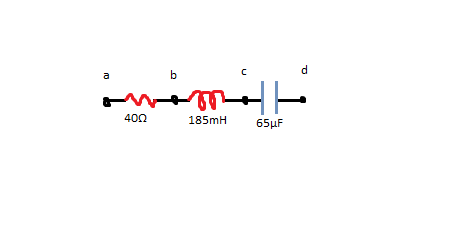# An AC source with \bigtriangleup V_{max} = 155 V and f = 70.0 Hz is connected between points a...

## Question:

An AC source with {eq}\bigtriangleup V_{max} {/eq} = 155 V and f = 70.0 Hz is connected between points a and d in the figure.a) Calculate the maximum voltages between points a and b.

b) Calculate the maximum voltages between points b and c.

c) Calculate the maximum voltages between points c and d.

d) Calculate the maximum voltages between points b and d.

## Impedance in LCR Series Circuits:

The RLC series circuit has a resistor, a capacitor and an inductor connected in series with an AC source.

The impedance of the resistor is given by the equation;

{eq}X_R = R = 40 \ \Omega {/eq}

The impedance of the inductor is given by the equation;

{eq}X_L = \omega L = 439.6 \times 185 \times 10^{-3} = 81.326 \ \Omega {/eq}

The impedance of the capacitor is given by the equation;

{eq}X_C = \dfrac{1}{\omega C} = \dfrac{1}{439.6 \times 65 \times 10^{-6}} = 34.996850283 \ \Omega {/eq}

The total impedance of the circuit is given by the equation;

{eq}Z = \sqrt{ R^2 + (X_C - X_L)^2} = \sqrt{ 40^2 + (34.996850283 - 81.326 )^2} = 61.207761873 \ \Omega {/eq}

where,

• {eq}\omega {/eq} is the angular frequency.
• R is the resistance of the resistor.
• L is the inductance of the inductor.
• C is the capacitance of the capacitor.

Maximum current

The maximum current can be obtained by using the equation;

{eq}I_{max} = \dfrac{V_{max}}{Z} {/eq}

where,

• {eq}V_{max} {/eq} is the maximum voltage.

Become a Study.com member to unlock this answer! Create your account

Given:

• An AC source with {eq}\Delta V_{max} = 150 \ V {/eq}
• Frequency of the AC source is {eq}f = 70.0 \ Hz {/eq}
• Resistance of a resistor is {eq}R...Impedance in Alternating Current Circuits

from

Chapter 13 / Lesson 8
4.1K

In this lesson, you will learn about topics that include the difference between DC and AC circuits, the use of rms values, definitions of impedance and reactance, and calculations involving the reactance of capacitors and inductors.